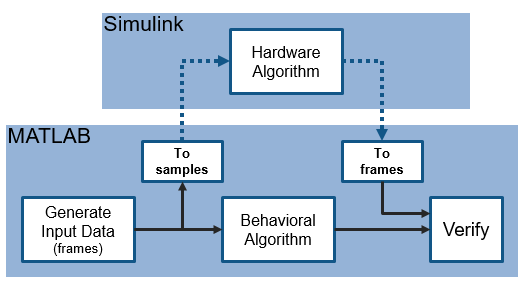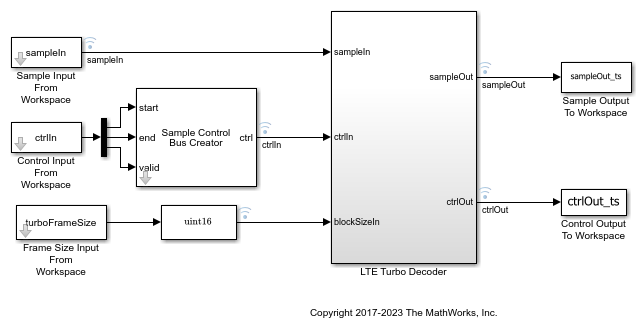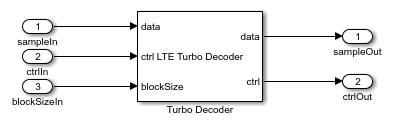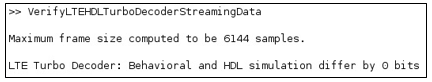Main Content

# Verify Turbo Decoder with Streaming Data from MATLAB

This example shows how to verify a hardware-targeted Turbo Decoder design using streaming data from MATLAB®.

To run this example, use the VerifyLTEHDLTurboDecoderStreamingData.m script.

LTE Toolbox™ and 5G Toolbox™ functions model operations on framed, floating-point and integer data and provide excellent behavioral references. Hardware designs must use streaming Boolean or fixed-point data. This example converts frames to samples in MATLAB and imports the sample stream to Simulink® for hardware algorithm design. The same data is applied to both the hardware algorithm in Simulink, and the behavioral algorithm in MATLAB. The output sample stream from the Simulink simulation is exported to MATLAB and then converted back to framed data for comparison.### Hardware Targeting in Simulink

The key features of a model for hardware targeting in Simulink® are:

• Streaming Sample Interface: LTE Toolbox and 5G Toolbox functions process frames while blocks in Wireless HDL Toolbox use a streaming sample interface. Serial processing is efficient for hardware designs. For further information, see Streaming Sample Interface. You can convert frames to samples in Simulink using the Frame To Samples block or in MATLAB using the `whdlFramesToSamples` function. In this example, we convert the frames to samples in MATLAB using the `whdlFramesToSamples` function.

• Subsystem Targeted for HDL Code Generation: Design a hardware-friendly sample-streaming model by selecting blocks from the Blocks. The part of the design targeted for HDL code generation must be in a separate subsystem.

• Conversion of Sample-Based Output to Frames: For verification, you can export the result of your hardware-compatible design to the MATLAB® workspace. You can then compare this result with the output of a MATLAB behavioral design. In this example, we convert the samples to frames in MATLAB using the `whdlSamplesToFrames` function.

You can use the VerifyLTEHDLTurboDecoderStreamingData.m MATLAB script to run the MATLAB behavioral code, set up, import data and run the Simulink™ model, export the data, and compare the behavioral and Simulink output.

The MATLAB script contains six parts:

1. Behavioral Simulation of Turbo Decoder

2. Conversion of Input Frames to Samples

3. Set Up the Simulink Model for Hardware Design

4. Run Simulink Model

5. Conversion of Output Samples to Frames

6. Verify Output of Simulink Model

### Behavioral Simulation of Turbo Decoder

For a behavioral simulation of the design, use the `lteTurboDecode` function from LTE Toolbox. The input to this function, softBits, is also the input for the HDL targeted design. The behavioral output of the `lteTurboDecode` function, rxBits can be used for comparison to the output of the HDL targeted design. Both softBits and rxBits are frames of floating-point data.

```% Turbo decoding of soft bits obtained from a noisy constellation turboFrameSize = 6144; txBits = randi([0 1],turboFrameSize,1); codedData = lteTurboEncode(txBits); txSymbols = lteSymbolModulate(codedData,'QPSK'); noise = 0.5*complex(randn(size(txSymbols)),randn(size(txSymbols))); rxSymbols = txSymbols + noise; scatter(real(rxSymbols),imag(rxSymbols),'co'); hold on; scatter(real(txSymbols),imag(txSymbols),'rx') legend('Rx constellation','Tx constellation') softBits = lteSymbolDemodulate(rxSymbols,'QPSK','Soft'); rxBits = lteTurboDecode(softBits); ```

### Conversion of Input Frames to Samples

First, convert the input frame to fixed-point.

```inframes = fi(softBits, 1, 5, 2); ```

Next, convert the framed data to a stream of samples and control signals using the `whdlFramesToSamples` function. This function also adds invalid samples to the sample stream to mimic streaming hardware data. The output of this function is the input to the Simulink model.

In this example, the `whdlFramesToSamples` function is configured to provide the inputs to the LTE Turbo Decoder block in the Simulink model. No invalid samples are inserted between valid samples.

The LTE Turbo Decoder block takes in a frame of data, one sample at a time, runs through the specified number of iterations and can then accept another input frame. To allow the block processing time to run the 6 iterations, we include invalid samples between frames. In this example, we set the number of invalid samples between frames to 7 iterations (or 14 half-iterations, each of which corresponds to a frame of delay). To calculate the exact cycles needed to process a frame, look at the LTE Turbo Decoder.

The turbo encoder function returns the systematic bits first, followed by the first set of parity bits and finally, the second set of parity bits. The LTE Turbo Decoder however requires each set of systematic and 2 parity bits to be sent in together. To reshape the input data to the Turbo Decoder block accordingly, we choose the option to compose the output samples from interleaved input samples.

```inframesize = 18444; % size of softBits ```
```% No invalid cycles between samples idlecyclesbetweensamples = 0; ```
```% Additional delay in frames for Turbo Decoder output numTurboIterations = 6; % approximate latency from Turbo Decoder block per half-iteration tdlatency = (ceil(turboFrameSize/32)+4)*32; % approximate delay in frames for Turbo Decoder output algframedelay = (2*numTurboIterations + 2)*(ceil(tdlatency/turboFrameSize)); idlecyclesbetweenframes = (inframesize/insamplesize)*algframedelay; ```
```% Output is composed of interleaved input samples % input: S_1 S_2 ... S_n P1_1 P1_2 ... P1_n P2_1 P2_2 ... P2_n % output: S_1 P1_1 P2_1 S2 P1_2 P2_2 ... Sn P1_n P2_n interleaveSamples = true; ```
```[sampleIn, ctrlIn] = ... whdlFramesToSamples(inframes, ... idlecyclesbetweensamples, ... idlecyclesbetweenframes, ... insamplesize, ... interleaveSamples); ```

### Set Up the Simulink Model for Hardware Design

The model imports sample-based data and control and the frame size from the MATLAB workspace.

```modelname = 'TurboDecoderStreamingDataHDLExample'; ```The LTE Turbo Decoder subsystem consists of the LTE Turbo Decoder block. This block is set to run 6 iterations of decoding.The MATLAB code defines the settings for the input size, sample time for the Simulink model, and computes the desired simulation time.

```% Settings for Signal From Workspace block samplesizeIn = 3; % encode rate is 1/3 ```
```sampletime = 1; ```
```simTime = size(ctrl,1); ```

### Run Simulink Model

You can run the model by clicking the Play button or calling the sim command on the MATLAB command line.

```sim(modelname); ```

### Conversion of Output Samples to Frames

Running the model results in streaming samples and control signals logged from the model in the variable sampleOut_ts and ctrlOut_ts. The sample and control data are converted to frames using the `whdlSamplesToFrames` function.

```% Reformat the logged data to form the sample and control output sampleOut = squeeze(sampleOut_ts.Data); ctrlOut = [squeeze(ctrlOut_ts.start.Data) ... squeeze(ctrlOut_ts.end.Data) ... squeeze(ctrlOut_ts.valid.Data)]; % Form frames from output sample and control data outframes = whdlSamplesToFrames(sampleOut, ctrlOut); % Gather all the bits - expecting only one frame rxBits_hdl = outframes{:}; ```

### Verify Output of Simulink Model

Compare the output of the HDL simulation to the output of the behavioral simulation to find the number of bit mismatches between the behavioral code and hardware-targeted model.

This simulation results in no bit errors. Increasing the amount of noise added to the samples or reducing the number of iterations may result in bit errors.

```% Check number of bit mismatches numBitsDiff = sum(rxBits_hdl ~= rxBits); fprintf(['\nLTE Turbo Decoder: Behavioral and ' ... 'HDL simulation differ by %d bits\n\n'], numBitsDiff); ```### Generate HDL Code and Verify its Behavior

Once your design is working in simulation, you can use HDL Coder™ to Generate HDL Code for the LTE Turbo Decoder subsystem. Use HDL Verifier™ to generate a SystemVerilog DPI Test Bench (HDL Coder) or run FPGA-in-the-Loop.

```makehdl([modelname '/LTE Turbo Decoder']) % Generate HDL code makehdltb([modelname '/LTE Turbo Decoder']) % Generate HDL Test bench ```

## Support

#### Deploying 5G NR Wireless Communications on FPGAs: A Complete MATLAB and Simulink Workflow

Download white paper